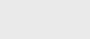3年前 (2020-10-11) |   抢沙发  690

## 递归算法示例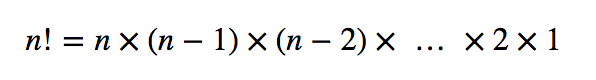``````public class Factorial {

// return n!
// precondition: n >= 0 and n <= 20
public static long factorial(long n) {
if      (n <  0) throw new RuntimeException("Underflow error in factorial");
else if (n > 20) throw new RuntimeException("Overflow error in factorial");
else if (n == 0) return 1;
else             return n * factorial(n-1);
}

public static void main(String[] args) {
long n = Long.parseLong(args);
StdOut.println(factorial(n));
}

}
``````

``````public static long factorial(int n) {
if (n == 1) return 1;
return n * factorial(n-1);
} ``````

``````factorial(5)
factorial(4)
factorial(3)
factorial(2)
factorial(1)
return 1
return 2*1 = 2
return 3*2 = 6
return 4*6 = 24
return 5*24 = 120``````

`factorial()`实现展示了每个递归函数所需的两个主要组件。

• 基本情况返回一个值，而不进行任何后续递归调用。对于一个或多个递归函数，可以对它求值。对于`factorial()`，基本情况为`n=1`
• 归约步骤是递归函数的核心部分。它将函数在一个（或多个）输入值处的值与函数在一个（或多个）其他输入值处的值相关联。此外，输入值的序列值必须收敛到基本情况。对于`factorial()`，每次调用n的值都会减少1，因此输入值序列会收敛到基本情况。

### 数学归纳法

• 基本情况：对于某些特定值或n的值（通常为0或1），证明语句为真。
• 归纳步骤：假设所有小于n的正整数的陈述都是真的，然后用这个事实来证明它对于n是真的。

### 欧几里得算法

``````public class Euclid {

// recursive implementation
public static int gcd(int p, int q) {
if (q == 0) return p;
else return gcd(q, p % q);
}

// non-recursive implementation
public static int gcd2(int p, int q) {
while (q != 0) {
int temp = q;
q = p % q;
p = temp;
}
return p;
}

public static void main(String[] args) {
int p = Integer.parseInt(args);
int q = Integer.parseInt(args);
int d  = gcd(p, q);
int d2 = gcd2(p, q);
StdOut.println("gcd(" + p + ", " + q + ") = " + d);
StdOut.println("gcd(" + p + ", " + q + ") = " + d2);
}
}
``````
``````gcd(1440, 408)
gcd(408, 216)
gcd(216, 192)
gcd(192, 24)
gcd(24, 0)
return 24
return 24
return 24
return 24
return 24 ``````

### 河内塔楼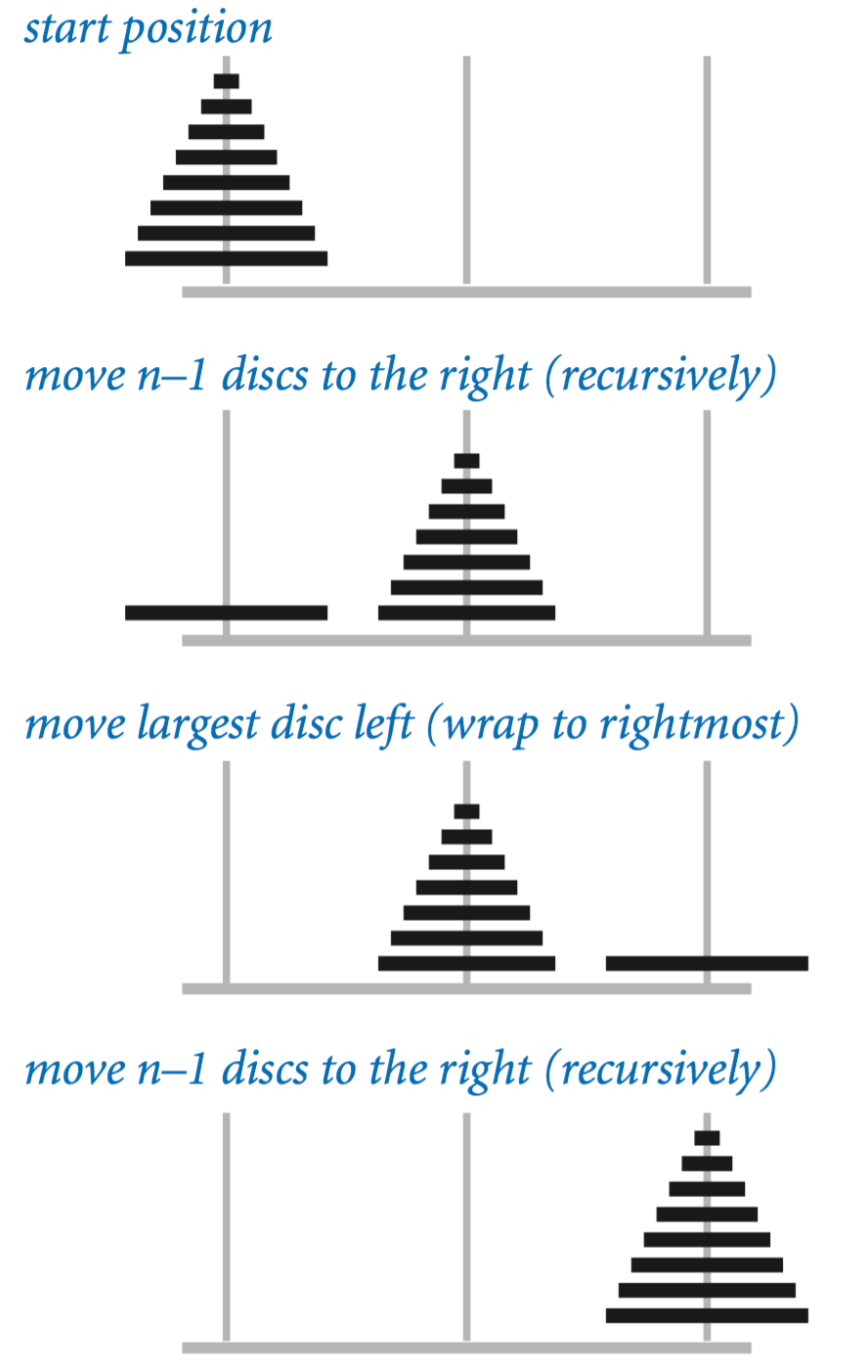• 一次只能移动一张光盘。
• 不要把大碟片放在小碟片上。
``````public class TowersOfHanoi {

// print out instructions for moving n discs to
// the left (if left is true) or right (if left is false)
public static void moves(int n, boolean left) {
if (n == 0) return;
moves(n-1, !left);
if (left) StdOut.println(n + " left");
else      StdOut.println(n + " right");
moves(n-1, !left);
}

public static void main(String[] args) {
int n = Integer.parseInt(args);
moves(n, true);
}

}
``````

### 指数时间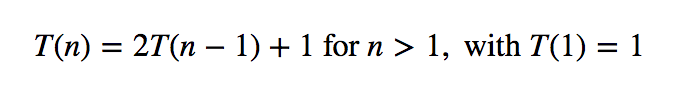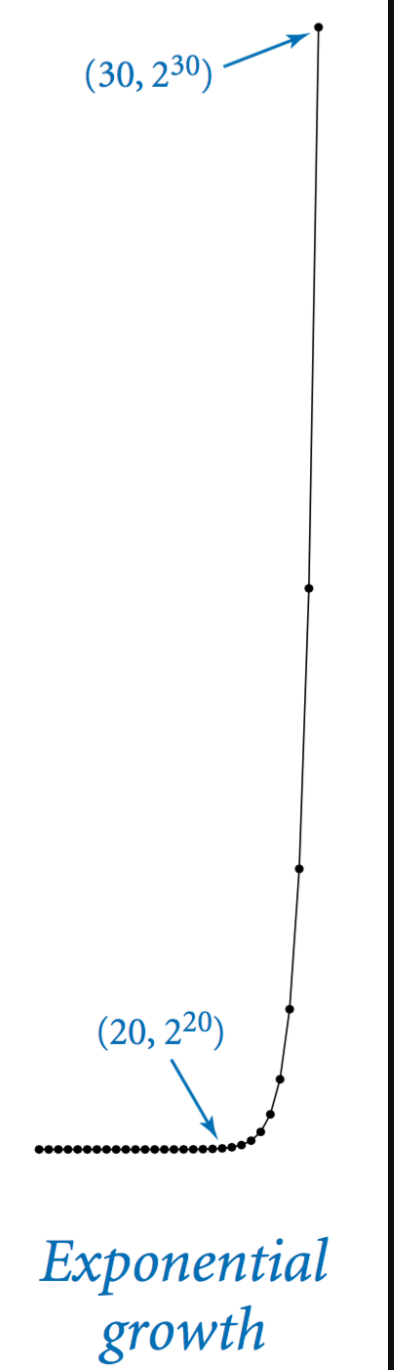### 灰色代码

n位格雷码是2n个不同的n位二进制数的列表，这样列表中的每个条目与它的前一个条目精确地相差一位。n位二进制反射格雷码递归定义如下：

• `n−1`位代码，每个字前加0，后跟
• 以相反顺序排列的`n−1`位代码，每个字前加1。

0位代码被定义为空，因此1位代码是0后跟1。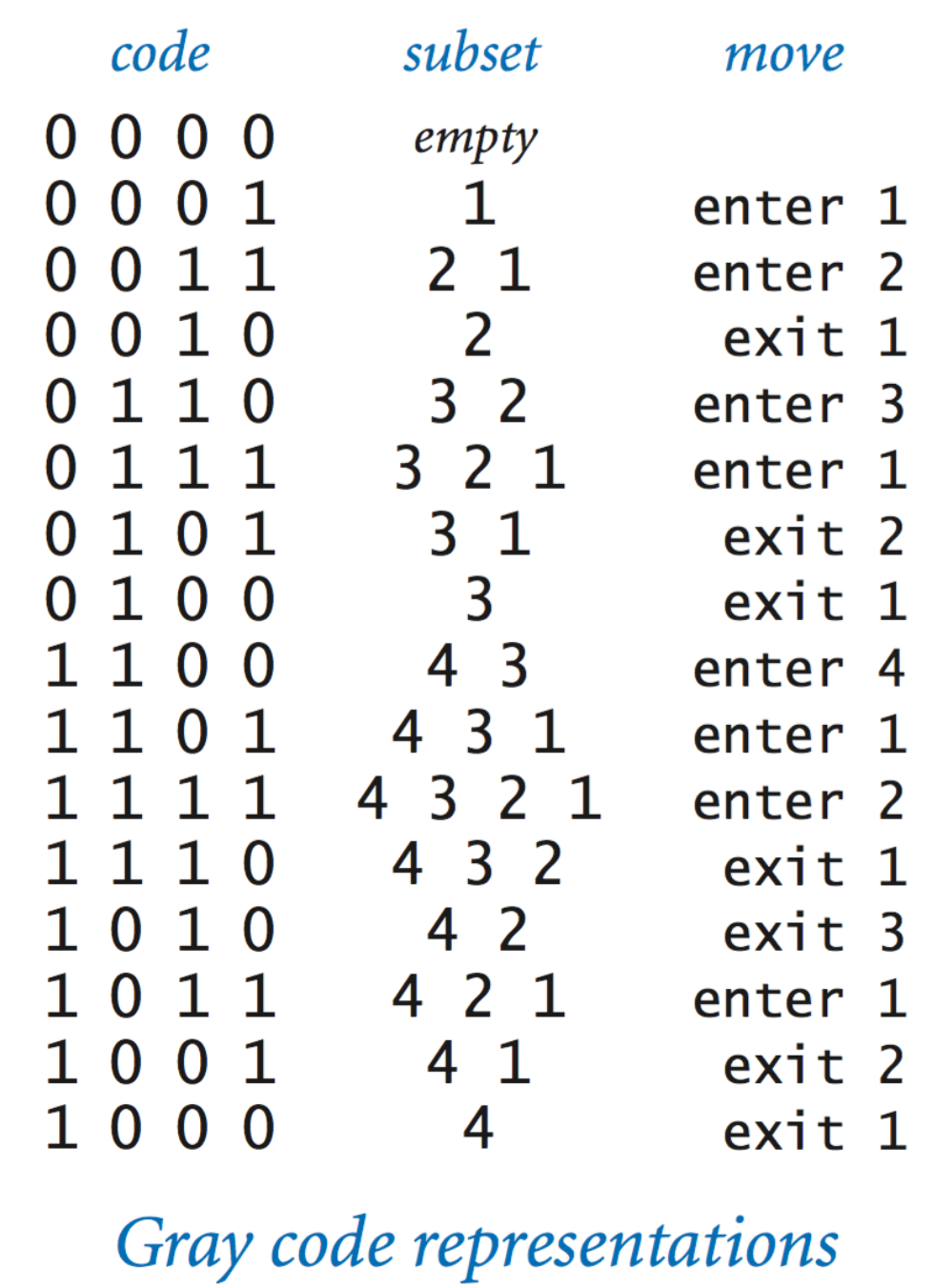``````public class Beckett {

public static void moves(int n, boolean forward) {
if (n == 0) return;
moves(n-1, true);
if (forward) StdOut.println("enter " + n);
else         StdOut.println("exit  " + n);
moves(n-1, false);
}

public static void main(String[] args) {
int n = Integer.parseInt(args);
moves(n, true);
}

}
``````

### 递归图形``````public class Htree {

// plot an H, centered on (x, y) of the given side length
public static void drawH(double x, double y, double size) {

// compute the coordinates of the 4 tips of the H
double x0 = x - size/2;
double x1 = x + size/2;
double y0 = y - size/2;
double y1 = y + size/2;

// draw the 3 line segments of the H
StdDraw.line(x0, y0, x0, y1);    // left  vertical segment of the H
StdDraw.line(x1, y0, x1, y1);    // right vertical segment of the H
StdDraw.line(x0,  y, x1,  y);    // connect the two vertical segments of the H
}

// plot an order n H-tree, centered on (x, y) of the given side length
public static void draw(int n, double x, double y, double size) {
if (n == 0) return;
drawH(x, y, size);

// compute x- and y-coordinates of the 4 half-size H-trees
double x0 = x - size/2;
double x1 = x + size/2;
double y0 = y - size/2;
double y1 = y + size/2;

// recursively draw 4 half-size H-trees of order n-1
draw(n-1, x0, y0, size/2);    // lower left  H-tree
draw(n-1, x0, y1, size/2);    // upper left  H-tree
draw(n-1, x1, y0, size/2);    // lower right H-tree
draw(n-1, x1, y1, size/2);    // upper right H-tree
}

// reads an integer command-line argument n and plots an order n H-tree
public static void main(String[] args) {
int n = Integer.parseInt(args);

double x = 0.5, y = 0.5;   // center of H-tree
double size = 0.5;         // side length of H-tree
draw(n, x, y, size);
}
}
``````### 注册